`COMPUTE! ISSUE 71 / APRIL 1986 / PAGE 42`

 Tug-A-War Mark Tuttle, Submissions Reviewer Don't be fooled by the apparent simplicity of this two-player strategy game. It looks easy on the surface, but it's a stiff test of your concentration and ability to think ahead. The original version was written for the Commodore 64. We've added new versions for the Atari 400/800, XL, and XE, Apple II-series computers, Atari 520ST, Amiga, IBM PC/PCjr, and the TI-99/4A. Since the game is based on colors, every version requires a color monitor or TV. The IBM version requires BASICA and a color/graphics adapter for the PC or Cartridge BASIC for the PCjr. The Atari version requires at least 16K of RAM, and the Amiga version requires at least 512K. Nearly everyone has played tug of war at one time or another. The traditional game pits two players or teams at opposite ends of a rope. At the middle of the rope is a flag, and each side tries to pull the flag into its territory. "Tug-a-War" is based on a similar concept. In this version, the flag is replaced with a round ball shape, and each player tries to maneuver the ball onto his or her side of the screen. Like many two-player games, the difficulty of Tug-a-War depends somewhat on the intelligence of your opponent. But even at the simplest level, you'll find that skill and foresight are essential to success.     Type in and save the appropriate program below. The rules are the same for every version (except Atari 520ST-see special instructions). Battle Of The Colors When you run Tug-a-War, two sets of colored boxes appear, one above the other. The lower, longer series of squares is the playing field. Near the middle of the playfield area is a round ball; the outermost boxes at each end of the playfield represent each player's home position. The players alternate turns, each trying to move the ball in their own direction, until it reaches one of the home squares.     So far, so good-but how do you move the ball? It's done not by pulling a rope, but by changing the colors of boxes in the playfield. The color of the square under the ball determines which direction it moves and how far it travels. On any given turn, the ball can move either one or two squares to the left, or one or two squares to the right. At the top of the screen are four boxes that show you which colors are linked to which directions. For instance, the leftmost box shows you which color makes the ball move one square to the left. The next box to the right shows you which color makes it move two squares to the left. The second pair of boxes show you which colors make the ball move in the opposite direction, to the right. By changing the color of the box where the ball is currently located, you can make it move toward your home square.     The playfield contains 11 boxes (9 in the TI version, 10 in the Atari ST version). When the game begins, each of these boxes is randomly given one of the four colors shown at the top of the screen. On each turn, you may change the color of one, several, or all of the boxes (however, you must always change at least one box). Below each box is a number which represents its distance from the home position of the player whose turn it is. For instance, if you are the player on the left, then on your turn the boxes are numbered 1, 2, 3, etc., from left to right (the tenth box is marked with a 0, and the eleventh with an A). When it's the right player's turn, the numbering is reversed (the rightmost box is 1, etc.).     To take a turn, you must select a number that corresponds to the numbers shown below the boxes in the playfield. This is done by pressing a single key. Press a number key from 1-0 to select one of the first ten values, or press the A key to choose the eleventh box. The number you choose determines how many boxes change color. For instance, if you press 1, only one box (the one nearest your home square) changes color. If you press 2, the two boxes nearest your home box change, and so on.     Where do the new colors come from? Every box cycles through the same series of four colors shown in the uppermost set of boxes, going from left to right. For example, if the colors shown there are white-blue-red-purple (the exact colors may be different on your computer), then a white square always changes to blue; a blue square always changes to red; a purple square changes to white, and so on. In other words, the box's current color determines which color it gets after the next color change.     Though every turn involves at least one color change, the ball doesn't necessarily move on every turn. It only moves when you change all the boxes between your home position and the current position of the ball. For example, if the ball is three boxes away from your home square, then you must change the color of at least three boxes in order to move it at all. Foresight Rewarded As you can see, there's much more to this game than appears on the surface. At first you might be tempted to try to move the ball as often as possible. But that's usually a losing strategy. Remember, the direction the ball moves depends on the color of its square before you take the turn.     In many cases, you'll want to move the ball only if it's on a color that moves it toward your goal. But like other games of strategy, Tug-aWar rewards the player who looks beyond the current move and tries to set things up for future moves; sometimes it's wise to make a small, temporary sacrifice in order to benefit later in the game. Because the boxes change colors in the same sequence, the effect of your own move is always completely predictable. However, since a single turn can change the color of many boxes, dramatic changes of fortune are also possible. Amiga And 520ST Versions Since the mouse is an integral system feature on both the Amiga and ST, both of these versions substitute mouse input for keyboard input. To select a square, simply move the mouse pointer to the desired box and press the left mouse button. Because keyboard prompts are unnecessary, no numbers are displayed below the playfield boxes.     Before entering BASIC to load the ST version, you should switch to the low-resolution graphics mode (use the Set Preferences option in the desktop's Options menu). Also, if your ST has 512K and a disk-based operating system, before running the program you should turn off buffered graphics (controlled by the Buffer Grph option in the Settings menu; it's off when no check appears beside the option in the menu). The standard 520ST leaves only about 5K free for BASIC programs, so Tug-a-War won't fit into memory unless the buffered graphics option is turned off. The program fits with buffered graphics switched on only if you have a 1040ST, or a 520ST with memory expansion, or a 520ST that has been upgraded with the TOS operating system in ROM chips (Read Only Memory).     The Amiga version uses the computer's built-in speech feature to announce the players' turns. In other respects, these games work exactly like the others. For instructions on entering these listings, please refer to "COMPUTE!'s Guide to Typing In Programs" in this issue of COMPUTE!.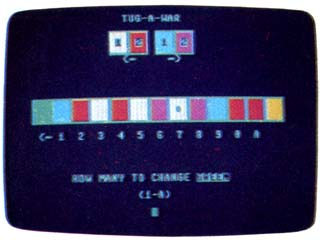"Tug-a-War" for the Commodore 64 and 128 is a game that looks simple, but demands good concentration and foresight. Program 1: Tug-A-War For Commodore 64/128 JJ 100 POKE53280,0:PRINT"{CLR}        ";:BC=53281:POKEBC,5:PC        (1)=5:PC(2)=7:PS=6:CR=1        :PL=1:X=20 CE 110 B=55715:A\$="O[]P{DOWN}        {3 LEFT}[] []{DOWN}        {3 LEFT}L[

]@":P\$(1)="G        REEN{RVS}{BLK} {OFF}        {LEFT}":P\$(2)="YELLOW":        B\$="2 UP}" DM 120 TM\$="{HOME}{9 DOWN}":Q\$        ="{RVS}{BLK}{34 SPACES}        ":DIMCL(11) QG 130 FORI=0TO23:PRINT"{RVS}        {BLK}{39 SPACES}" KE 140 POKE1063+(40*I),160:POK        E55335+(40*I),0:NEXT AQ 150 PRINT"{RVS}{39 SPACES}        {HOME}":POKE2023,160:PO        KE56295,0 DG 160 PRINT"{HOME}"SPC(15)"        {RVS}TUG-A-WAR":PRINTSP        C(13)"{DOWN}{RVS}{WHT}"        A\$B\$"{RED}"A\$B\$"{BLK}        {CYN}"A\$B\$"{PUR}"A\$B\$ RS 170 PRINTSPC(14)"{BLK}1"SPC        (2)"2"SPC(3)"1"SPC(2)"2        {DOWN}":PRINTSPC(15)"        {RVS}" HK 180 GOSUB620:PRINTTM\$"        {DOWN}{RVS} {GRN}"A\$B\$;        :FORZ=1TO11:Y=INT(4*RND        (1))+1:CL(Z)=Y:POKE646,        Y FQ 190 PRINT"{RVS}"A\$B\$;:NEXTZ       :PRINT"{YEL}{RVS}"A\$ CB 210 POKE646,PEEK(B)AND15:PR        INTTM\$"{2 DOWN}"SPC(X)"        {RVS}Q":POKEBC,PC(PL) SF 220 PRINTTM\$"{14 DOWN}"SPC(        18)"{RVS}{BLK}        {3 SPACES}" HR 230 AN=0:PRINTTM\$SPC(7)"        {10 DOWN}{BLK}{RVS}HOW        {SPACE}MANY TO CHANGE        {OFF}"P\$(PL) MA 240 PRINTSPC(17)"{DOWN}        {RVS}(1-A){2 DOWN}        {3 LEFT}[]{LEFT}"; BR 250 POKE204,0:POKE198,0:WAI        T198,1:GETMT\$ RB 260 IFASC(MT\$)<48ORASC(MT\$)        <>65ANDASC(MT\$)>57THEN2        50 XR 270 POKE204,1:IFMT\$="A"THEN        AN=11:MT\$="{LEFT}ALL":G        OTO300 CR 280 IFMT\$="0"THENAN=10:MT\$=        "10":GOTO300 HC 290 AN=VAL(MT\$) KM 300 PRINT"{2 LEFT}        {5 SPACES}{3 LEFT}"MT\$ ?FS 310 IFAN<1ORAN>11THEN220 FF 320 IFPL=2THENAN=12-AN:GOTO        440 HE 330 IFAN11THENPL=2:WC=7:B=        55734:X=38:GOTO530 KD 420 IFPL=ITHENPL=2:GOSUB640        :GOSUB630:GOTO210 HE 430 PL=1:GOSUB640:PRINT"        {10 UP}":GOSUB620:GOTO2        10 XH 440 FORQ=ANTO11 DH 450 IFAN<1ORAN>11THEN220 BD 460 IFAN>PSTHENCK=1 GJ 470 IFCL(Q)=4THENCL(Q)=1:GO        TO360 HB 480 CL(Q)=CL(Q)+1:GOTO360 JX 490 B=B+6:X=X+6:PS=PS+2:RET        URN PA 500 B=B-3:X=X-3:PS=PS-1:RET        URN XM 510 B=B-6:X=X-6:PS=PS-2:RET        URN BE 520 B=B+3:X=X+3:PS=PS+1:RET        URN FF 530 POKE646,PEEK(B)AND15:PR        INT"{HOME}{11 DOWN}"SPC        (X)"{RVS}Q":PRINTTM\$"        {10 DOWN}; PG 540 FORE=1TO5:PRINTQ\$:NEXT:        GOSUB640:GOSUB640 FM 550 PRINT"{HOME}{ll DOWN}"S        PC(11)P\$(PL)" IS THE WI        NNER":Z=WC:FORI=1TO11:P        OKEBC,Z CP 560 IFZ=0THENZ=WC:GOSUB610:        NEXT SJ 570 Z=0:GOSUB610:NEXT BP 580 POKEBC,15:PRINTTM\$SPC(1        1)"{10 DOWN}{RVS}LIKE T        O PLAY AGAIN{2 DOWN}        {11 LEFT}{RVS}Y/N" RA 590 POKE198,0:WAIT198,1:GET        MT\$:IFMT\$<>"N"THENRUN JC 600 POKE198,0:SYS198 QC 610 FORP=1TO200:NEXTP:RETUR        N CG 620 PRINTTM\$"{5 DOWN}{RVS}        {BLK}{2 SPACES}":RETURN AQ 649 PRINTTM\$"{5 DOWN}{RVS}        {BLK}{39 SPACES}":RETUR        N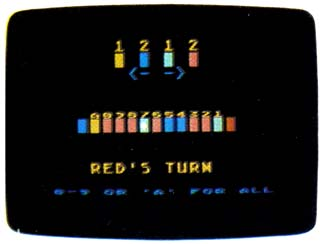This version of "Tug-a-War" runs on all Atari 400, 800, XL, and XE computers. Program 2: Tug-A-War For Atari 400/800, XL, XE Version by Kevin Mykytyn, Editorial Programmer Blue indicates inverse video OC 10 CB=PEEK(106)-8:POKE 10       6,CB-4:GRAPHICS 18:DIM        C\$(4),COL(11),MOV(4),       K\$(1):C\$="ZzzZ" BI 20 MOV(1)=-1:MOV(2)=-2:MO       V(3)=1:MOV(4)=2:OPEN #       1,4,8,"K:":GOSUB 190:G       OSUB 250 KL 30 BP=6: PL=0 AP 40 PL=(PL=0):GOSUB 290:PO       KE 53248,72+8*BP NK 50 GET #1,K:K\$=CHR\$(K):IF        K\$="A" THEN SP=11:GOT        O 90 GB 60 IF K\$="0" THEN SP=10:G       OTO 90 HF 70 IF K\$<"1" OR P:\$>"9" TH       EN 50 OL 80 SP=VAL(K\$) CK 90 IF (PL=0 AND 12-SP>BP)        OR (PL=1 AND SP12 THEN BP=12 HM 110 IF BP=-1 THEN BP=0 KI 120 GOSUB 320 LK 130 IF BP>0 AND BP<12 THE        N 40 MG 140 POKE 53248,72+8*BP:PO        SITION 4,9 EN 150 IF BP=0 THEN PRINT #6        ;" BLUE WINS! ":GOTO        170 DJ 160 PRINT #6;" RED WINS!        " CI 170 POSITION 0,11:PRINT #        6;"{3 SPACES}press an        y key{3 SPACES}" BK 180 GET #1,K:GOTO 30 JH 190 NS=CB*256:POSITION 5,        5:PRINT #6;"PLEASE WA        IT" NF 200 FOR A=0 TO 1023:POKE        NS+A,PEEK(57344+A):NE        XT A FC 210 POKE 756,CB:FOR A=NS+        464 TO NS+471:POKE A,        126:NEXT A CO 220 FOR A=NS+1536 TO NS+1        920:POKE A,0:NEXT A:F        OR A=NS+1596 TO NS+16        03:POKE A+256,126:POK        E A+128,126:NEXT A BI 230 POKE 559,46:POKE 623,        1:POKE 53250,72:POKE        53249,168:POKE 53277,        3:POKE 54279,CB+4:POK        E 704,15:POKE 705,52 JB 240 FOR A=NS+1598 TO NS+1        601:READ B:POKE A,B:N        EXT A:POKE 706,132:RE        TURN :DATA 24,60,60,2        4 FP 250 PRINT #6;"{CLEAR}":PO        SITION 6,0:PRINT #6;"        1 2 1 2":POSITION 6,1        :PRINT #6;"Z Z z Z":P        OSITION 7,2:PRINT #6;        "<- ->" DJ 260 GB=PEEK(560)+256*PEEK        (561):POKE GB+10,6:PO        KE GB+16,6 KL 270 POSITION 4,6:FOR A=1        TO 11:Q=INT(RND(1)*4+        1):COL(A)=Q:PRINT #6;        C\$(Q,Q);:NEXT A IM 280 POSITION 0,11:PRINT #        6;" 0-9 OR 'A' FOR AL        L" :RETURN KJ 290 POSITION 4,9 FG 300 IF PL=1 THEN PRINT #6        ;"BLUE'S TURN":POSITI        ON 4,5:FOR A=1 TO 9:P        RINT #6;A;:NEXT A:PRI        NT #6;"0A":RETURN BH 310 PRINT #6;"RED'S TURN        ":POSITION 4,5:PRINT        #6;"A0";:FOR A=9 TO 1         STEP -1:PRINT #6;A;:        NEXT A:RETURN PD 320 IF PL=0 THEN 340 JI 330 FOR A=1 TO SP:GOSUB 3        50:NEXT A:RETURN PE 340 FOR A=11 TO 12-SP STE        P -1:GOSUB 350:NEXT A        :RETURN LH 350 COL(A)=COL(A)+1-4*(CO        L(A)=4):POSITION 3+A,        6:PRINT #6;C\$(COL(A),        COL(A)):RETURN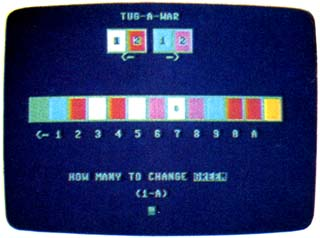"Tug-a-War" for the IBM PC and PCjr. Program 3: Tug-A-War For IBM PC/PCjr Version by Kevin Mykytyn, Editorial Programmer GE 10 GOTO 30 GN 20 FOR ROW=0 TO 2:LOCATE Y+RO       W,PS*3+X:PRINT B\$:NEXT:RET       URN NO 30 KEY OFF:SCREEN 0,0:WIDTH 4       0:B\$=CHR\$(222)+CHR\$(219)+C       HR\$(221)+CHR\$(31) HP 40 DIM COL(11):BP=6:C(1)=2:C(       2)=6:C(3)=3:C(4)=5:PL=0:RA       NDOMIZE TIMER LA 50 MOV(1)=-1:MOV(2)=-2:MOV(3)       =1:MOV(4)=2 LP 60 GOSUB 190 CI 70 PL=(PL=0):GOSUB 230:GOSUB       240:GOSUB 180 LA 80 K\$=INKEY\$:IF K\$="a" OR K\$=       "A" THEN SP=11:GOTO 110 0J 90 IF K\$="0" THEN SP=10:GOTO       110 HE 100 IF K\$<"1" OR K\$>"9" THEN        80 ELSE SP=VAL(K\$) HG 110 IF (PL=0 AND 12-SP>BP) OR         (PL=-1 AND SP        12 THEN BP=12 ELSE IF BP=        -1 THEN BP=0 NO 130 GOSUB 260:IF BP>0 AND BP<        12 THEN 70 LJ 140 GOSUB 230:LOCATE 20,15:CO        LOR 9:IF BP=0 THEN PRINT        " Blue Wins! ":GOTO 160 GL 150 COLOR 4:PRINT " Red Wins!          " FA 160 LOCATE 23,8:COLOR 14:PRIN        T "Press any key to play        again":GOSUB 180 GJ 170 A\$=INKEY\$:IF A\$="" THEN 1        70 ELSE RUN FF 180 DEF SEG=0:POKE 1050,PEEK(        1052):RETURN BE 190 CLS:Y=3:X=0:FOR A=1 TO 4:        PS=4+A:COLOR C(A):GOSUB 2        0:NEXT FE 200 COLOR 14:PRINT SPC(15)CHR        \$(17)" "STRING\$(2,17)" "        CHR\$(16)"  "STRING\$(2,16) EI 210 Y=13:X=1:COLOR 9:PS=0:GOS        UB 20:FOR A=1 TO 11:Q=INT        (RND(1)*4+1):COL(A)=Q:COL        OR C(Q):PS=A:GOSUB 20:NEX        T:COLOR 4:PS=12:GOSUB 20 IL 220 LOCATE 23,8,0:COLOR 10:PR        INT "Press (0-9) or `A' f        or all":RETURN GF 230 COLOR 15:LOCATE 14,BP*3+2        :PRINT CHR\$(219):RETURN BG 240 LOCATE 20,15:IF PL=0 THEN         COLOR 4:PRINT "Red's Tur        n ":LOCATE 11,5:PRINT "A         0 ";:FOR A=9 TO 1 STEP-1        :PRINT A;:NEXT:RETURN LF 250 COLOR 9:PRINT "Blue's Tur        n":LOCATE 11,4:FOR A=1 TO         9:PRINT A;:NEXT:PRINT "        0 A":RETURN HI 260 IF PL=0 THEN 280 BO 270 FOR A=1 TO SP:GOSUB 290:N        EXT:RETURN FM 280 FOR A=11 TO 12-SP STEP-1:        GOSUB 290:NEXT:RETURN DE 290 COL(A)=COL(A)+1+4*(COL(A)        =4):COLOR C(COL(A)):PS=A:        GOSUB 20:RETURN Program 4: Tug-A-War For Apple Version by Tim Victor, Editorial Programmer 3B 100 GOSUB 400 8A 110 HGR : HOME SC 120 HCOLOR= 3: FOR TD = - 1 T        O 1 STEP 2: FOR TN = 0 TO         1: FOR TX = - 1 TO TN ST        EP 2: GOSUB 500: NEXT : N        EXT : NEXT DC 130 VP = 40 A1 140 FOR I = 0 TO 3:HC = CT(I)        :PS = 4.5 + I: GOSUB 430:         NEXT E8 150 VP = 146:HC = 1:PS = 0: G        OSUB 430: GOSUB 460 AE 160 FOR I = 0 TO 10:BC(I) = I        NT ( RND (1) * 4):HC = CT        (BC(I)):PS = I + 1: GOSUB        430: NEXT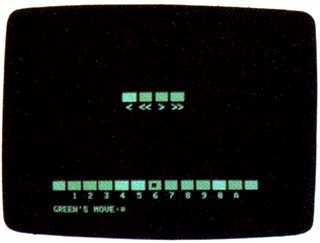Apple "Tug-a-War," a challenging strategy game. C9 170 HC = 6:PS = 12: GOSUB 430        : GOSUB 460 F9 180 BP = 5: GOSUB 470 69 190 VTAB 21: FOR I = 1 TO 11:         HTAB I X 3 + 2: IF I < 1         0 THEN PRINT CHR\$ (48 + I         ); 89 200 IF I = 10 THEN PRINT "0"; 15 210 IF I = 11 THEN PRINT "A"; E4 220 NEXT : VTAB 23: HTAB 1: P        RINT "GREEN'S MOVE:"; 46 230 GOSUB 520:A = A - 1: IF (        BP < = A) THEN BP = BP +        JT(BC(BP)) 97 240 FOR I = 0 TO A:BC(I) = BC        (I) + 1 - 4 * (BC(I) = 3)        :HC = CT(BC(I)):PS = I +        1: GOSUB 430: NEXT 54 250 GOSUB 470 16 260 IF BP < 0 OR BP > 10 THEN         360 67 270 VTAB 21: FOR I = 1 TO 11:         HTAB (12 - I) * 3 + 2: I        F I < 10 THEN PRINT CHR\$        (48 + I); 99 280 IF I = 10 THEN PRINT "0"; 25 290 IF I = 11 THEN PRINT "A"; 80 300 NEXT : VTAB 23: HTAB 1: P        RINT "BLUE'S MOVE: "; 1F 310 GOSUB 520:A = 11 - A: IF        (BP > = A) THEN BP = BP +         JT(BC(BP)) 5A 320 FOR I = 10 TO A STEP - 1:        BC(I) = BC(I) + 1 - 4 * (        BC(I) = 3):HC = CT(BC(1))        :PS = I + 1: GOSUB 430: N        EXT 51 330 GOSUB 470 13 340 IF BP < 0 OR BP > 10 THEN         360 9F 350 GOTO 190 92 360 PS = 12 * (BP > 0) - 1: H        COLOR= 4 * (BP > 0): GOSU        B 490 33 370 VTAB 23: HTAB 1: IF BP <        0 THEN PRINT "GREEN WINS          ": GOTO 390 6C 380 IF BP > 10 THEN PRINT "BL        UE WINS    " 8A 390 GET A\$: GOTO 110 DE 400 FOR I = 0 TO 3: READ CT(I        ): NEXT 3B 410 FOR I = 0 TO 3: READ JT(I        ): NEXT : RETURN 84 420 DATA 3,5,6,2,-1,-2,1,2 8C 430 HCOLOR= HC: FOR YP = VP T        O VP + 10 9A 440 HPLOT PS * 21 + 1,YP TO P        S * 21 + 17,YP: NEXT IE 450 RETURN AB 460 HCOLOR= 3: FOR YP = VP +        1 TO VP + 9 STEP 2: HPLOT         PS * 21 + 1,YP TO PS * 2        1 + 17,YP: NEXT : RETURN 52 470 IF BP < 0 OR BP > 10 THEN         RETURN 19 480 HCOLOR= 4 * (CT(BC(BP)) >         3):PS = BP 74 490 FOR YP = VP + 3 TO VP + 7        : HPLOT PS * 21 + 27,YP T        O PS * 21 + 32,YP: NEXT :         RETURN DF 500 TP = 124 + (TD + TN) * 21         + TN * TX * 4:TL = TP +        TO * 3:TR = TP - TD * 3 E5 510 HPLOT TR,60 TO TL,57 TO T        R,54: RETURN 86 520 POKE 49168,0: GET A\$: IF        A\$ = CHR\$ (3) THEN END B9 530 IF A\$ = CHR\$ (3) THEN END CB 540 IF A\$ < > "A" AND A\$ < >        "a" AND (A\$ < "0" OR A\$ >         "9") THEN 520 36 550 IF A\$ = "A" OR A\$ = "a" T        HEN A\$ = CHR\$ (59) 38 560 IF A\$ = "0" THEN A\$ = CHR        \$ (58) 51 570 A = ASC (A\$) - 48: RETURN Program 5: Tug-A-War For Atari ST Version by Kevin Mykytyn, Editorial Programmer 10 fullw 2:clearw 2:color 1,1,1 20 bp=6:c(1)=6:c(2)=7:c(3)=10:c(4)=12:pl    =0 30 mov(1)=-l:mov(2)=-2:mov(3)=1:mov(    4)=2 40 gosub 170:gosub 270 50 pl=(pl=0):gosub drawball:gosub play    er 60 gosub readmouse:if y<98 or y>127 o    r x<34 or x>273 then 60 70 sp=int((x-11)/24) 80 if (pl=0 and sp>bp) or (pl=-1 and sp    11 the      n bp=11 else if bp=-l then by=0 110 gosub colchange 120 gosub drawball:if bp>0 and bp" 460 FOR I=1 TO 15 470 PRINT 480 NEXT I 490 FOR I=1 TO 9 500 RANDOMIZE 510 KHAR(I)=INT(4*RND)+1 520 NEXT I 530 FOR R=13 TO 15 540 CALL HCHAR(R,2,96,2) 550 FOR I=1 TO 9 560 KH=96+KHAR(I)*8 570 CALL HCHAR(R,I*3+1,KH) 580 CALL HCHAR(R,I*3+2,KH) 590 CALL HCHAR(R,I*3+3,KH+1     ) 600 NEXT I 610 CALL HCHAR(R,31,136,2) 620 NEXT R 630 CALL HCHAR(14,BP,96+KHA     R(PS)18+2) 640 IF (PS=0)+(PS=10)THEN 1     180 650 A\$=Y\$(PL) 660 R=17 670 C=1 680 GOSUB 110 690 CALL HCHAR(24,17,32) 700 A\$=P\$(PL)&"'S TURN " 710 R=20 720 C=11 730 GOSUB 110 740 R=22 750 C=14 760 A\$="(1-9)" 770 GOSUB 110 780 GOSUB 1020 790 CALL KEY(0,K,H) 800 IF H=0 THEN 790 810 IF (K<49)+(K>57)THEN 79     0 820 AN=K-48 830 CALL HCHAR(24,17,K) 840 IF PL=0 THEN 890 850 AN=10-AN 860 S=AN 870 E=9 880 GOTO 910 890 S=1 900 E=AN 910 GOSUB 1100 920 FOR Q=S TO E 930 IF KHAR(Q)<>4 THEN 960 940 KHAR(Q)=1 950 GOTO 970 960 KHAR(Q)=KHAR(Q)+1 970 NEXT Q 980 PL=-(PL=0) 990 GOTO 530 1000 CALL COLOR(9,1,5) 1010 CALL COLOR(14,1,7) 1020 FOR I=1 TO 8 1030 CALL COLOR(I,PC(PL),2) 1040 NEXT I 1050 CALL COLOR(10,PC(PL),1      6) 1060 CALL COLOR(11,PC(PL),1      1) 1070 CALL COLOR(12,PC(PL),8      ) 1080 CALL COLOR(13,PC(PL),1      4) 1090 RETURN 1100 IF ((ANPS)*(PL=1)THEN 1170 1110 A=(KHAR(PS)=1)+(KHAR(P      S)=2)*2-(KHAR(PS)=3)-(      KHAR(PS)=4)*2 1120 BP=BP+A\$3 1130 PS=PS+A 1140 IF (PS>0)*(PS<10)THEN      1170 1150 PS=-(PS=-1)+(PS=11)+PS 1160 BP=-(BP<5)*3-(BP>29)*3      1 1170 RETURN 1180 R=14 1190 C=7 1200 A\$=P\$(-(PS=10))&" IS T      HE WINNER!" 1210 GOSUB 110 1220 A\$="LIKE TO PLAY AGAIN       (Y/N)?" 1230 R=24 1240 C=4 1250 GOSUB 110 1260 CALL KEY(0,K,H) 1270 IF H=0 THEN 1260 1280 IF (K<>78)*(K<>89)THEN      1260 1290 IF K=89 THEN 330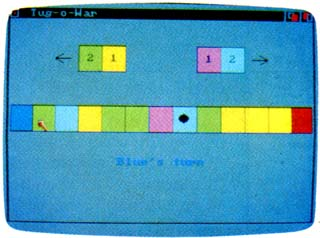This version of "Tug-a-War" uses several of the Amiga's 4,096 different color shades. Program 7: Tug-A-War For Amiga Version by John Krause, Assistant Technical Editor SAY TRANSLATE\$("")← SCREEN 2,320,200,3,1← WINDOW 2," Tug-A-War "„12,2← FOR i=0 TO 7←   READ r,g,b←  PALETTE i,r,g,b← NEXT← RANDOMIZE TIMER← DIM a(11)← FOR i=1 TO 11←   a(i) =INT(RND(1)*4)+4← NEXT← row=3← co1=3:colr=4:GOSUB frame:GOSUB squ are← co1=4:colr=5:GOSUB frame:GOSUB squ are← cot=8:colr=6:GOSUB frame:GOSUB squ are← co1=9:colr=7:GOSUB frame:GOSUB squ are← row=10← LOCATE 5,11:COLOR 1,4:PRINT "2"← LOCATE 5,14:COLOR 1,5:PRINT "1"← LOCATE 5,26:COLOR 1,6:PRINT "1"← LOCATE 5,29:COLOR 1,7:PRINT "2"← LINE (84,36)-STEP(-16,0),1← LINE -STEP(8,4),1← LINE (48,36)-STEP(8,-4),1← LINE (248,36)-STEP(16,0),1← LINE -STEP(-8,4),1← LINE (264,36)-STEP(-8,-4),1← FOR col=0 TO 12←   GOSUB frame← NEXT← col=0:colr=3:GOSUB square← co1=12:colr=2:GOSUB square← dot=6:GOSUB update← SAY TRANSLATE\$("welcome to tug o wa r.")← main:← LOCATE 17,15← IF red THEN←  COLOR 2,0:PRINT "Red's turn "←  SAY TRANSLATE\$("reds turn.")← ELSE←   COLOR 3,0:PRINT "Blue's turn"←  SAY TRANSLATE\$("blues turn.")← END IF WHILE MOUSE(0)<>1 OR MOUSE(4)<8 0 OR MOUSE(4)>104 OR MOUSE(3)<2 3 OR MOUSE(3)>276← WEND← click= INT(MOUSE(3)/24)← IF (red AND click<=dot) OR (red=0 AN D click>=dot) THEN ←   temp=dot←   IF a(temp)=4 THEN dot=dot-2←  IF a(temp)=5 THEN dot=dot-1←  IF a(temp)=6 THEN dot=dot+1←  IF a(temp)=7 THEN dot=dot+2← END IF← IF red THEN←   FOR i=click TO 11←   a(i)=a(i)+1←   IF a(i)=8 THEN a(i)=4←  NEXT ELSE←   FOR i=1 TO click←   a(i)=a(i)+1←   IF a(i)=8 THEN a(i)=4←   NEXT← END IF← IF dot>11 THEN←  dot=12:GOSUB update←  LOCATE 17,15:COLOR 2,0:PRINT " Re d wins! "←  SAY TRANSLATE\$("red wins.")←  GOTO quit← END IF← IF dot<1 THEN←  dot=0:GOSUB update←  LOCATE 17,15:COLOR 3,0:PRINT "Blu e wins! "←  SAY TRANSLATE\$("blue wins.")←  GOTO quit← END IF← GOSUB update← red=1-red← GOTO main← frame:← x=24:IF 24*col>280 THEN x=23← LINE (24*col,8*row)-STEP(x,24),l,b← RETURN← square:← x=22:IF 24*col+1>280 THEN x=21← LINE (24*col+1,8*row+1)-STEP(x,22),c olr,bf← RETURN← update:← FOR col=1 TO 11←  colr=a(col):GOSUB square← NEXT← CIRCLE (24*dot+11,91),5,1← PAINT (24*dot+11,91),1← RETURN← quit:← LOCATE 19,7:COLOR 1,0:PRINT "Clic k mouse to play again."← SAY TRANSLATE\$("click mouse to pla y again.")← WHILE MOUSE(0)=0:WEND← RUN← DATA .5,.5,.5,0,0,0,1,0,0,0,0,1,0,1,0,1,1, 0,1,0,1,0,1,1←